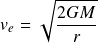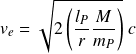# Escape velocity

Escape velocity tells us the strength of a gravitational field in terms of velocity. The formula quantifies the velocity at which the kinetic energy of a second body will overcome the gravitational field produced by a first body.

The strength of a gravitational field is determined by the mass density operator, which gives the ratio of length to mass in a region of space. Since the mass density operator acts on both parts of the 2-part energy mechanism (wavelength and velocity), the square root of the operator is taken along with a constant of 2.

The mass density operator requires ratios of length and mass to normalize the intensive quantity of mass density over the corresponding volume, where the Planck scale serves as the basis.

## Historical formula

The elementary formula for escape velocity is derived from the historical formula by replacing the gravitational constant with elementary units of length, mass, and time. The formularestated in natural units isThe elementary form of the equation gives the operator and the maximum velocity potentialEscape velocity